Next: Exercises Up: Two-Dimensional Compressible Inviscid Flow Previous: Linearized Supersonic Flow

# Flat Lifting Wings

In Section 15.9, we found that the pressure coefficients on the upper and lower surfaces of a thin, flat airfoil of infinite span, inclined at a small angle of attack,, to uniform supersonic flow of Mach number, are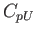(15.196)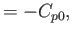(15.197)

respectively, where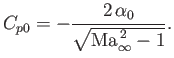(15.198)

Of course, a realistic airfoil has a finite span. The aim of this section is to investigate the effect of finite span on the lift developed by a flat airfoil.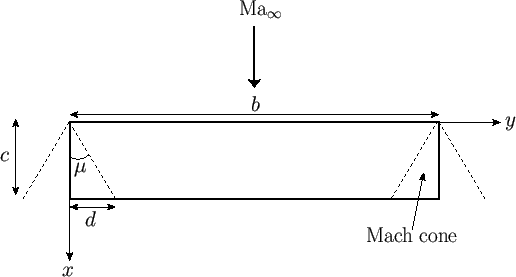Suppose that the airfoil is rectangular, with span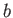, and width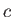, as shown in Figure 15.13. The unperturbed flow is parallel to the-axis, and the airfoil is inclined at a small angleto the plane. Because of the limited regions of influence in supersonic flow, the fact that the span is finite is only felt within the Mach cones emanating from the two leading-edge corners of the airfoil. This implies that the two-dimensional results (15.196) and (15.197) are applicable everywhere on the airfoil surface, except inside the two Mach cones. In other words, the inner part of the airfoil, lying between the two cones, behaves as if it were part of an airfoil of infinite span.

Assuming that the left-most leading-edge corner lies at the origin, the pressure coefficient on the upper surface of the airfoil in the left-most Mach cone can be shown to take the form (Milne-Thompson 1958)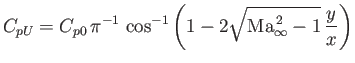(15.199)

forand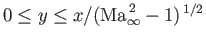. The corresponding pressure coefficient on the lower surface of the airfoil is written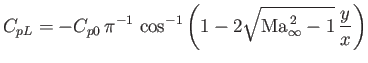(15.200)

forand. There are analogous expressions for the pressure coefficients in the right-most Mach cone. The variation of the pressure coefficient along the span of a finite, flat airfoil is illustrated in Figure 15.14.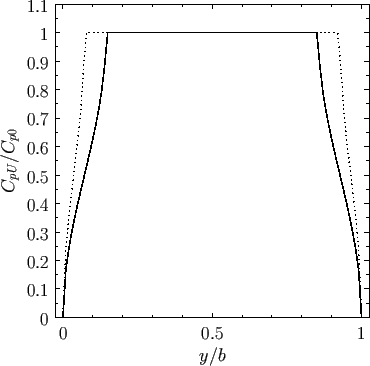Note that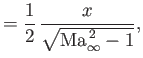(15.201)

which implies that the average pressure coefficient inside the two Mach cones on the upper surface of the airfoil is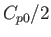. Similarly, the average pressure coefficient inside the Mach cones on the lower surface is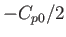. Thus, the coefficient of lift of the whole airfoil is less than the two-dimensional value,, by an amount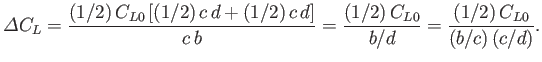(15.202)

The terms in the denominator are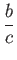(15.203)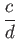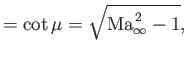(15.204)

where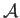is termed the aspect-ratio of the airfoil. The previous three equations can be combined to give(15.205)

where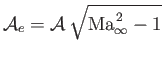(15.206)

is termed the effective aspect-ratio of the airfoil. Figure 15.15 shows the loss of lift of a flat airfoil as a function of the effective aspect-ratio. Expression (15.205) is valid for values ofas small as unity (which corresponds to the case where the Mach cones emanating from each leading-edge corner are just beginning to intersect the opposite side of the airfoil). For lower values of, the expression is different, but tends to zero, as indicated by the broken lin in the figure.Next: Exercises Up: Two-Dimensional Compressible Inviscid Flow Previous: Linearized Supersonic Flow
Richard Fitzpatrick 2016-03-31